# Fat 4-polytopes

 Importance: High ✭✭✭
 Author(s): Eppstein, David Kuperberg, Greg Ziegler, Gunter M.
 Subject: Geometry » Polytopes
 Keywords: f-vector polytope
The fatness of a 4-polytope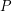is defined to bewhere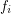is the number of faces ofof dimension.
Question   Does there exist a fixed constantso that every convex 4-polytope has fatness at most?
The-vector of a-dimensional polytopeis the vector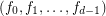whereis the number of faces of dimension. Let us denote by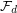the collection of all-vectors of convex-dimensional polytopes. Steinitz proved that the setis completely characterized by the following three conditions:
\item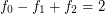, \item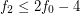, \item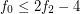.
In sharp contrast to this, the situation forseems to be quite complicated. For instance, it has been shown thatdoes not contain all elements ofwhich lie in the convex hull of; i.e.,has "holes" in it. For the extreme examples of simple and simplicial polytopes, the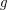-theorem of Billera-Lee and Stanley gives a complete description of all possible-vectors, but in general very little is known.